Electron.n. J. Diff. Eqns. Vol. 1993(1993), No. 02, pp. 1-10.

### A singular perturbation problem in integrodifferential equations James H. Liu

Abstract:
Consider the singular perturbation problem for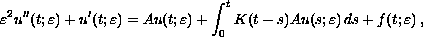where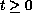,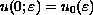,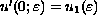, and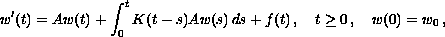in a Banach space X when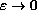. Here A is the generator of a strongly continuous cosine family and a strongly continuous semigroup, and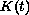is a bounded linear operator for. With some convergence conditions on initial data and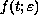and smoothness conditions on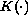, we prove that when, one hasand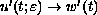in X uniformly on [0,T] for any fixed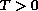. An application to viscoelasticity is given.

Submitted June 14, 1993. Published September 16, 1993.
Math Subject Classification: 45D, 45J, 45N.
Key Words: Singular perturbation, Convergence in solutions and derivatives.

Show me the PDF file (139 KB), TEX file, and other files for this article.

James H. Liu
Department of Mathematics, James Madison University
Harrisonburg, VA 22807, USA
e-mail: liu@math.jmu.edu